# COMPLIANCE WITH BASEL III AND BASEL IV FRAMEWORK

The following provides a quick summary of Basel III and Basel IV compliance when using the IRM methodology:

• Figure 3.11 shows Monte Carlo risk simulations applied to determine confidence levels, percentiles, and probabilities of occurrence using historically fitted data or forecast  These methods are in line with Basel III and Basel IV requirements Sections 16 and 161 concerning the use of historical simulations, Monte Carlo simulations, and 99th percentile confidence intervals.
• Figure 3.12 shows a correlated simulation of a portfolio of assets and liabilities, where asset returns are correlated against one another in a portfolio and optimization routines were run on the simulated results. These processes provide compliance with Basel III and Basel IV requirements Sections 178, 232, and 527(f) involving correlations, Value at Risk (VaR) models, portfolios of segments, and pooled exposures (assets and liabilities).
• Figure 3.13 shows Value at Risk percentile and confidence calculations using structural models and simulation results that are in line with Basel III and Basel IV requirements Sections 179, 527(c), and 527(f).
• Figure 3.14 shows the computations of the probability of default(PD) as required in the Basel Accords, specifically Basel III and Basel IV Section 733 and Annex 2’s Section 16. PD can be computed using structural models or based on historical data through running basic ratios to more advanced binary logistic models.
• Figure 3.15 shows the simulation and generation of interest rate yield curves using Risk Simulator and Modeling Toolkit models. These methods are in line with Basel III and Basel IV requirements Section 763 requiring the analysis of interest rate fluctuations and interest rate shocks.
• Figure 3.16 shows additional models for volatile interest rates, financial markets, and other liquid instruments’ instantaneous shocks using Risk Simulator’s stochastic process models. These analyses conform to Basel III and Basel IV requirements Sections 155, 527(a), and 527(b).
• Figure 3.17 shows several forecast models with high predictive and analytical power, which is a part of the Risk Simulator family of forecast methods. Such modeling provides compliance with Basel III and Basel IV requirements Section 417 requiring models of good predictive power.
• Figure 3.18 shows the list of financial and credit models available in the ROV Modeling Toolkit and ROV Real Options SLS software applications. These models conform to Basel III and Basel IV requirements Sections 112, 203, and 527(e) requiring the ability to value and model over-the-counter (OTC) derivatives, nonlinear equity derivatives and convertibles, hedges, and embedded options.
• Figure 3.19 shows the modeling of foreign exchange instruments and hedges to determine the effectiveness of foreign exchange hedging vehicles and their impact on valuation, portfolio profitability, and VaR, in line with Basel III and Basel IV Sections 131 and 155 requiring the analysis of different currencies, correlations, volatility, and hedges.
• Figure 3.20 shows the option-adjusted spread (OAS), credit default swaps (CDS), and credit spread options (CSO) models in ROV Modeling Toolkit. These models provide compliance with Basel III and Basel IV requirements Sections 140 and 713 pertaining to modeling and valuing credit derivatives and credit hedges.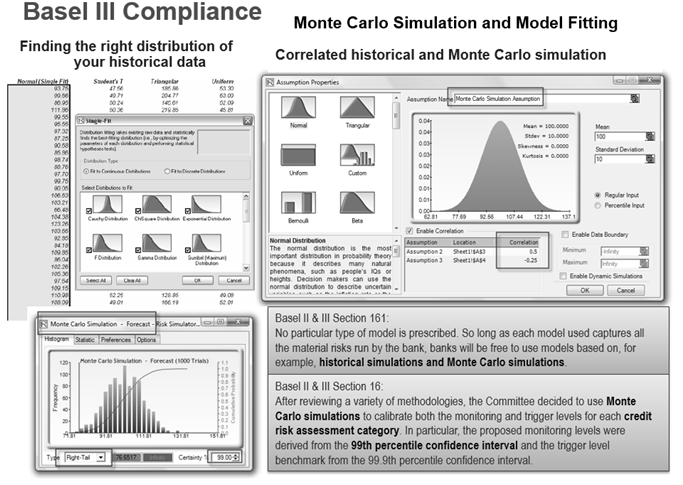Figure 3.11: Basel III and Basel IV Confidence Levels, Monte Carlo Simulations, and Credit Risk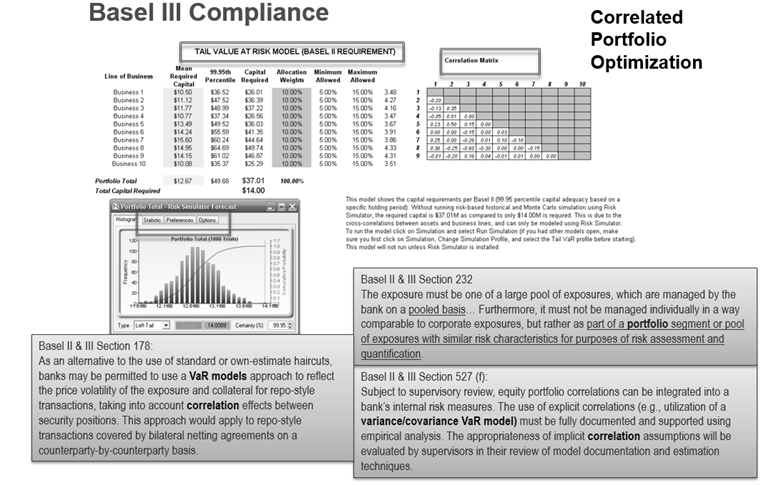Figure 3.12: Basel III and Basel IV Correlated Portfolios and Correlated Simulations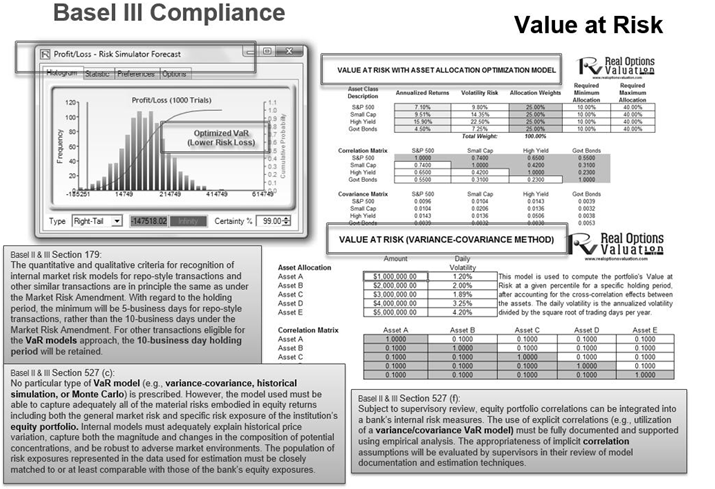Figure 3.13: Basel III and Basel IV Value at Risk and Percentiles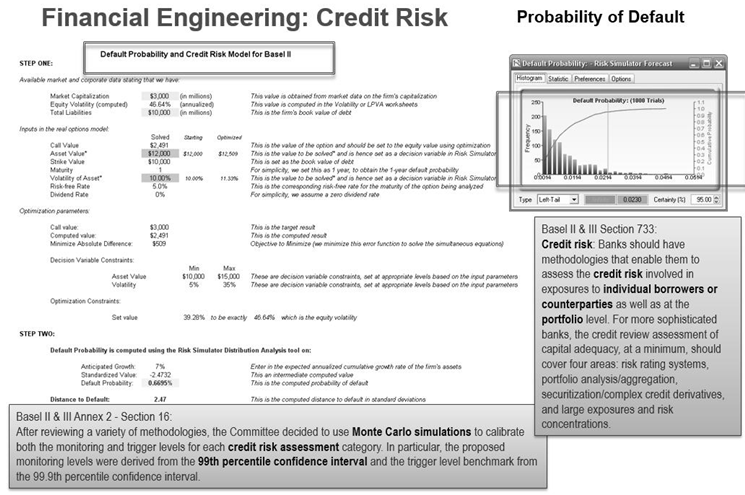Figure 3.14: Basel III and Basel IV Credit Risk Analysis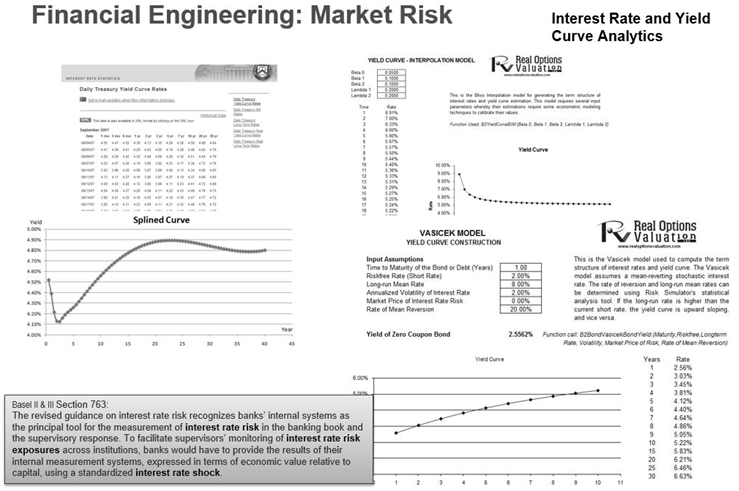Figure 3.15: Basel III and Basel IV Interest Rate Risk and Market Shocks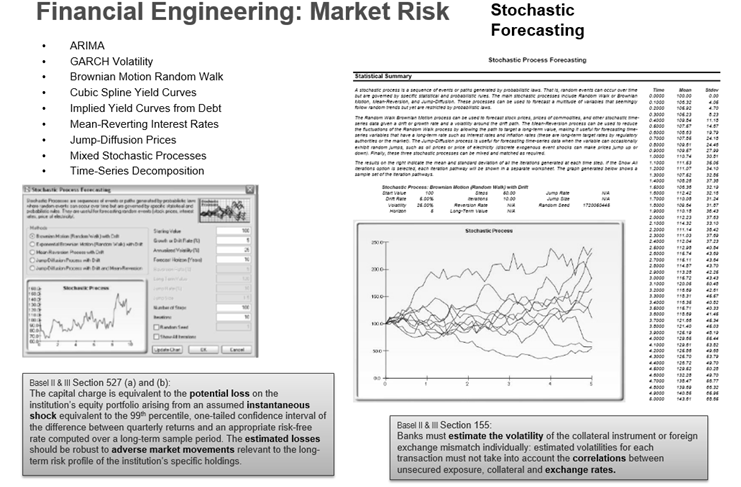Figure 3.16: Basel III and Basel IV Volatility and Adverse Instantaneous Shocks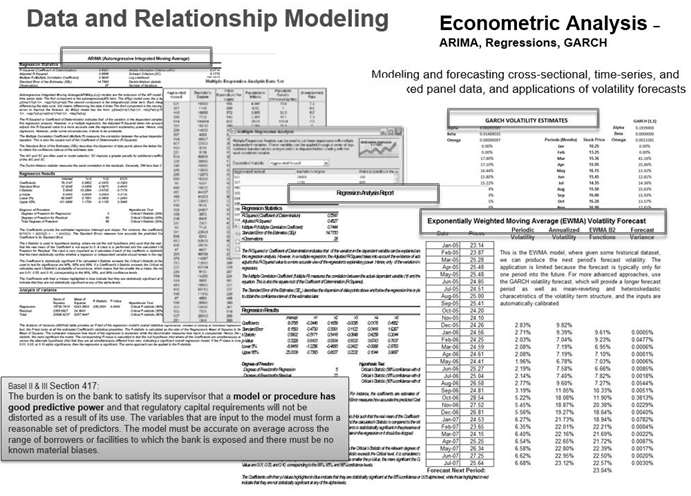Figure 3.17: Basel III and Basel IV Forecast Models with Strong Predictive Power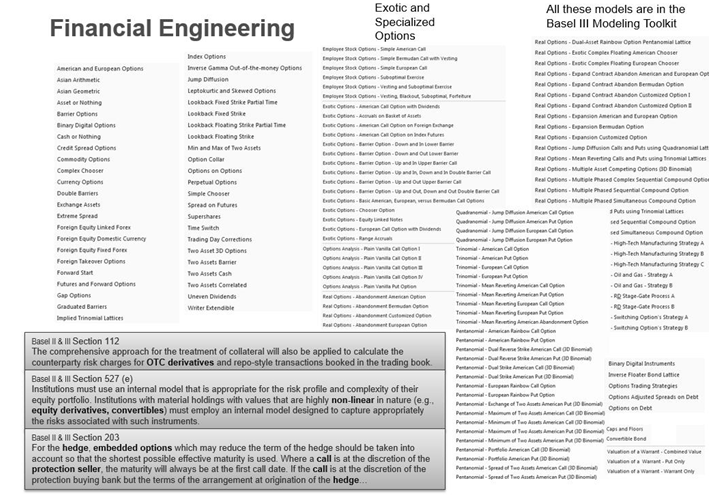Figure 3.18: Basel III and Basel IV Modeling OTC Derivatives and Exotic Convertibles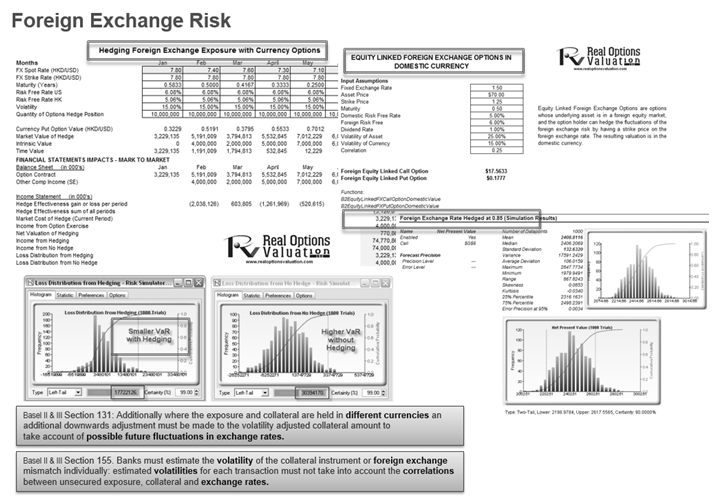Figure 3.19: Basel III and Basel IV Modeling Foreign Exchange FluctuationsFigure 3.20: Basel III and Basel IV Credit Derivatives and Hedging

error: Content is protected !!